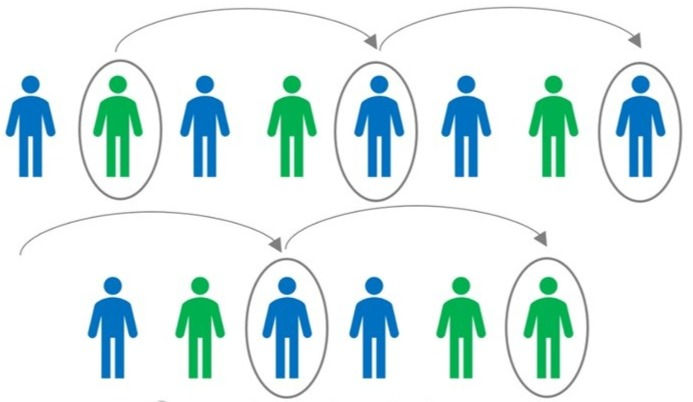top of page
Search

# What is sampling?

### What are the kinds of sampling, and why is it so important to planners?

Surveys are a method of collecting primary data, ie, crowd sourcing data from relevant populations. A sample is a representative of a larger population, and is mostly used in surveys where the entire population cannot be surveyed effectively. This saves time and cost involved in large scale surveying.

For any sample to be a valid representation of a population, it has to fulfil certain criteria. There is where terms like confidence levels and error margins are used, But more on that later, first let's check out how do you even select a sample number, and how do you go about choosing the right kind of sampling method for your survey.

There are 2 major types - Probabilistic and Non-probabilistic, based on whether any degree of probability is used in the sample selection or not.#### Probabilistic sampling methods

##### A - Random Sampling

In simple random sample, every person from a given population is at equal chance of being selected to be surveyed. Mostly carried out using random number generation, this is the easiest form.

For example, in a given neighborhood, all houses are to be numbered, and using a random number generator on the internet, a few numbers can be selected, and only those houses will be surveyed as a sample for the entire neighborhood.##### B - Cluster Sampling

In this method, the overall population is divided into significant clusters based on characteristics like age, sex, migration, employment etc. This makes the survey more relevant to the questions being asked.

For example, social media surveys are usually conducted on populations regularly using their phones. This sharply reduces the sample to only the relevant population.##### C- Systematic Sampling

Similar to random sampling, the population is numbered but the sample is selected at regular intervals. For example, in a neighborhood of a 100 houses, the researcher may choose to survey every 10th house, ie, 1, 11, 21, 31 etcD - Stratified Random Sampling

In this type, the population is divided into stratas, and sampling is done within each strata. For example, selecting set number of samples within each income range, or within each age range. The entire population is surveyed, but is classified by one characteristic.### Where does this help?

Probabilistic methods create accurate samples and reduce the risk of bias in research. While slightly difficult to conduct as the researcher has no way to influence the study, it is a more acceptable sampling method to conduct. It also gives a good overview of wide demographical answers.

#### Non- Probabilistic sampling methods

##### A - Convenience Sampling

Convenience samples are drawn as per the researcher's convenience. Google form surveys are mostly seen as a form of convenience sampling as it can be sent to whoever the researcher deems as a good sample.

##### B - Snowball Sampling

Similar to convenience sampling, this method depends on using one sample to get more survey samples. For example, getting contacts of more experts from one of your interviewees for thesis is a form of snowball sampling.

##### C - Purposive Sampling

In this method, samples are rejected based on their answers, and only those fulfilling the criteria of the surveyor are retained. For example, in a survey of body washes, the surveys answering that they do not use body washes are rejected from any further study.

##### D - Quota Sampling

This is similar to stratified sampling, but here the samples within each strata are not selected using any probabilistic method.

##### E - Voluntary Sampling

In this, only those who wish to be surveyed as included as samples. This is majorly used in marketing studies.Taken from Scribbr. com.

### Where does this help?

These methods are used when no prior information is available to make assumptions. It helps in creating a problem statement and direction of research.

Although at risk of bias, these methods are easier for the researcher, and save on time and cost.

### So how do you decide which to pick?

This 100% depends on your research domain and question. Are you conducting a demography survey? Random sampling? Do you have very specific questions that require prior expertise to answer? Purposive or Snowball sampling may help. The onus is on the researcher to prove that their methods are suited to the research question that they wish to answer.

### Ok great...So how do I calculate the sample size?

Cochran's formula is used to calculate the sample size of populations in simple random sampling, and is widely used in Urban Planning for demographic sample size calculation.Where:

• e is the the margin of error

• p is the (estimated) proportion of the population which has the attribute in question,

• q is 1 – p.

• Z value is taken from a Z value table available online (Google it!)

### But wait, what does this mean?

In any survey, as it is a sample and not the entire population, there is bound to be a degree of difference from an actual whole population survey. This precision is known as the confidence of the survey. For example, in a 95% confident survey, if the survey is repeated with different samples, the answers will remain the same 95% of the time.

This means the accepted margin for error is 5%, or 0.05. In case it is not specified, p and q are taken as 0.5 each. This is based on the Z curve. The Z value is of the confidence level. Look up the Z value of 95%, that is the input in the formula.

There are many modifications to this formula depending on size of the population, error margins and so on. Here is the link to an online sample size calculator.

A/N - I hope this has been a simple and easy introduction to surveying and sampling. Do drop a message if you have any doubts or queries!